×#### Thank you for registering.

One of our academic counsellors will contact you within 1 working day.

Click to Chat

1800-1023-196

+91-120-4616500

CART 0

• 0

MY CART (5)

Use Coupon: CART20 and get 20% off on all online Study Material

ITEM
DETAILS
MRP
DISCOUNT
FINAL PRICE
Total Price: Rs.

There are no items in this cart.
Continue Shopping• Complete JEE Main/Advanced Course and Test Series
• OFFERED PRICE: Rs. 15,900
• View Details

```Chapter 17: Understanding Shapes III Special Types of Quadrilaterals Exercised – 17.1

Question: 1Given below is a parallelogram ABCD. Complete each statement along with the definition or property used.

(ii) ∠DCB =

(iii) OC =

(iv) ∠DAB + ∠CDA =

Solution: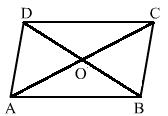The correct figure is

(i) AD = BC (opposite sides of a parallelogram are equal)

(ii) ∠DCB = ∠BAD (opposite angles are equal)

(iii) OC = OA (diagonals of a parallelogram bisect each other)

(iv) ∠DAB + ∠CDA = 180° (the sum of two adjacent angles of a parallelogram is 180°)

Question: 2

The following figures are parallelograms. Find the degree values of the unknowns x, ySolution:

(i) Opposite angles of a parallelogram are same.

Therefore, x = z and y = 100°

Also, y + z = 180° (sum of adjacent angle of quadrilateral is 180°)

z + 100° = 180°

x = 180° – 100°

=> x = 80°

Therfore, x = 80°, y = 100° and z = 80°

(ii) Opposite angles of a parallelogram are same.

Therefore, x = y and ∠ROP = 100°

∠PSR + ∠SRQ = 180°

=> y + 50° = 180°

x = 180° – 50°

=> x = 130°

Therefore, x=130°, y=130°

Since y and z are alternate angles, z = 130°.

(iii) Sum of all angles in a triangle is 180°

Therefore, 30° + 90° +z = 180°

=>z = 60°

Opposite angles are equal in the parallelogram.

Therefore, y = z = 60°and x = 30° (alternate angles)

(iv) x = 90° (vertically opposite angle)

Sum of all angles in a triangle is 180°.

Therefore, y + 90° + 30° = 180°

=> y=180° - (90° + 30°)

=> y = 60°

y = z = 60° (Alternate Angles)

(v) Opposite angles are equal in a parallelogram.

Therefore, y = 80°

y + x = 180°

=> x = 180° – 100° = 80°

z = y = 80° (alternate angles)

(vi) y = 112° (opposite angles are equal in a parallelogram)

In triangle UTW:

x + y + 40° = 180° (angle sum property of a triangle)

x = 180° – (112° – 40°) = 28°

Bottom left vertex = 180° – 112° = 68°

Therefore, z = x = 28° (alternate angles)

Question: 3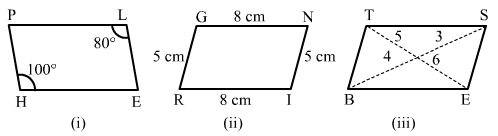Solution:

(i) No. This is because the opposite angles are not equal.

(ii) Yes. This is because the opposite sides are equal.

(iii) No. This is because the diagonals do not bisect each other.

Question: 4

In the adjacent figure HOPE is a parallelogram. Find the angle measures x, y and z. State the geometrical truths you use to find them.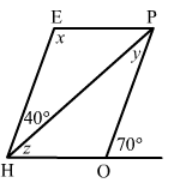Solution:

∠H0P + 70° = 180° (linear pair)

∠HOP = 180° – 70° =110°

x = ∠HOP = 110° (opposite angles of a parallelogram are equal)

∠EHP + ∠HEP = 180° (sum of adjacent angles of a parallelogram is 180°)

110° + 40° + z = 180°

z = 180° – 150° = 30°

y = 40° (alternate angles)

Question: 5

In the following figures GUNS and RUNS are parallelograms. Find x and y.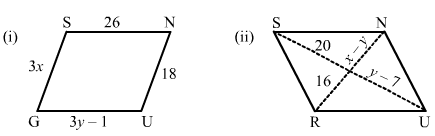Solution:

(i) Opposite sides are equal in a parallelogram.

Therefore, 3y – 1 = 26

=> 3y = 27

y = 9.

Similarly, 3x = 18

x = 6.

(ii) Diagonals bisect each other in a parallelogram.

Therefore, y – 7 = 20

y = 27

x – y = 16

x – 27 = 16

x = 43.

Question: 6

In the following figure RISK and CLUE are parallelograms. Find the measure of x.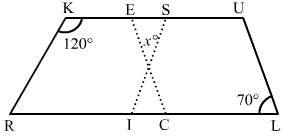Solution:

In the parallelogram RISK:

∠ISK + ∠RKS = 180° (sum of adjacent angles of a parallelogram is 180°)

∠ISK = 180° – 120° = 60°

Similarly, in parallelogram CLUE:

∠CEU = ∠CLU = 70° (opposite angles of a parallelogram are equal)

In the triangle:

x + ∠ISK + ∠CEU = 180°

x = 180° – 70° + 60°

x = 50°.

Question: 7

Two opposite angles of a parallelogram are (3x – 2)° and (50 – x)°. Find the measure of each angle of the parallelogram.

Solution:

Opposite angles of a parallelogram are congruent.

Therefore, 3x – 2° = 50 – x°

3x° – 2° = 50° – x°

3x° + x° = 50° + 2°

4x° = 52°

x° = 13°

Putting the value of x in one angle:

3x° – 2° = 39° – 2° = 37°

Opposite angles are congruent.

Therefore, 50° – x° = 37°

Let the remaining two angles be y and z.

Angles y and z are congruent because they are also opposite angles.

Therefore, y = z

The sum of adjacent angles of a parallelogram is equal to 180°

Therefore, 37° + y = 180°

y = 180° – 37°

y = 143°

So, the angles measure are: 37°, 37°, 143° and 143°.

Question: 8

If an angle of a parallelogram is two-third of its adjacent angle, find the angles of the parallelogram.

Solution: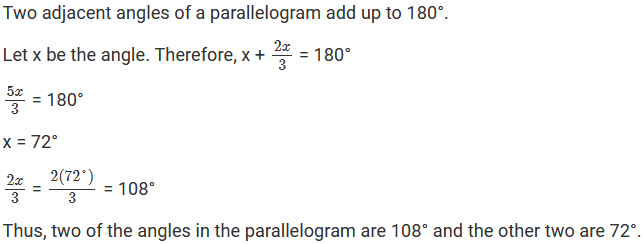Question: 9

The measure of one angle of a parallelogram is 70°. What are the measures of the remaining angles?

Solution:

Given that one angle of the parallelogram is 70°.

Since opposite angles have same value, if one is 70°, then the one directly opposite will also be70°

So, let one angle be x°.

x° + 70° = 180° (the sum of adjacent angles of a parallelogram is 180°)

x° = 180° – 70°

x° = 110°

Thus, the remaining angles are 110°, 110° and 70°.

Question: 10

Two adjacent angles of a parallelogram are as 1: 2. Find the measures of all the angles of the parallelogram.

Solution:

Let the angle be A and B.

The angles are in the ratio of 1: 2.

Measures of ∠A and ∠B are x° and 2x°.

Then, As we know that the sum of adjacent angles of a parallelogram is 180°.

Therefore, ∠A + ∠B = 180°

=>x° + 2x° = 180°

=> 3x° = 180°

=> x° = 60°

Thus, measure of ∠A = 60°, ∠B = 120°, ∠C = 60° and ∠D = 120°.

Question: 11

In a parallelogram ABCD, ∠D = 135°, determine the measure of ∠A and ∠B.

Solution:

In a parallelogram, opposite angles have the same value.

Therefore, ∠D = ∠B = 135°

Also, ∠A + ∠B +∠C + ∠D = 360° and ∠A + ∠D = 180°.

∠A = 180° – 135° = 45°.

Question: 12

ABCD is a parallelogram in which ∠A = 70°. Compute ∠B, ∠C and ∠D.

Solution:

Opposite angles of a parallelogram are equal.

Therefore, ∠C = 70° = ∠A

∠B = ∠D

Also, the sum of the adjacent angles of a parallelogram is 180°

Therefore, ∠A + ∠B = 180°

70° + ∠B = 180°

∠B = 110°

∠C = 70°

∠D = 110°

Question: 13

The sum of two opposite angles of a parallelogram is 130°. Find all the angles of the parallelograms.

Solution:

Let the angles be A, B, C and D.

It is given that the sum of two opposite angles is 130°.

Therefore, ∠A + ∠C = 130°

∠A + ∠A = 130° (opposite angles of a parallelogram are equal)

∠A = 65°and ∠C = 65°

The sum of adjacent angles of a parallelogram is 180°.

∠A + ∠B =180°

65° + ∠B = 180°

∠B = 180° – 65°

∠B = 115°

Therefore, ∠A = 65°, ∠B = 115°, ∠C = 65° and ∠D = 115°.

Question: 14

All the angles of a quadrilateral are equal to each other. Find the measure of each. Is the quadrilateral a parallelogram? What special type of parallelogram is it?

Solution:

Let the angle be x.

All the angles are equal.

Therefore, x + x + x + x = 360°.

4x = 360°.

x = 90°.

So, each angle is 90° and quadrilateral is a parallelogram. It is a rectangle.

Question: 15

Two adjacent sides of a parallelogram are 4 cm and 3 cm respectively. Find its perimeter.

Solution:

We know that the opposite sides of a parallelogram are equal.

Two sides are given, i.e. 4 cm and 3 cm. Therefore, the rest of the sides will also be 4 cm and 3 cm.

Therefore, Perimeter = Sum of all the sides of a parallelogram = 4 + 3 + 4 + 3 = 14 cm

Question: 16

The perimeter of a parallelogram is 150 cm. One of its sides is greater than the other by 25 cm. Find the length of the sides of the parallelogram.

Solution:

Opposite sides of a parallelogram are same.

Let two sides of the parallelogram be x and y.

Given: x = y + 25

Also, x + y + x + y = 150 (Perimeter= Sum of all the sides of a parallelogram)

y + 25 + y + y + 25 + y = 150

4y = 150 – 50

4y = 100

y = 100/4 = 25

therefore, x = y + 25 = 25 + 25 = 50

Thus, the lengths of the sides of the parallelogram are 50 cm and 25 cm.

Question: 17

The shorter side of a parallelogram is 4.8 cm and the longer side is half as much again as the shorter side. Find the perimeter of the parallelogram.

Solution:

Given:

Shorter side = 4.8 cm, Longer side = 4.8/2 + 4.8 = 7.2 cm

Perimeter = Sum of all sides = 4.8 + 4.8 + 7.2 + 7.2 = 24 cm

Question: 18

Two adjacent angles of a parallelogram are (3x – 4)° and (3x + 10)°. Find the angles of the parallelogram.

Solution:

We know that the adjacent angles of a parallelogram are supplementary.

Hence, 3x + 10° and 3x – 4° are supplementry.

3x + 10° + 3x – 4° = 180°

6x° + 6° = 180°

6x° = 174°

x = 29°

First angle = 3x + 10° = 3(29°) + 10° = 97°

Second angle = 3x – 4° = 83°

Thus, the angles of the parallelogram are 97°, 83°, 97° and 83°

Question: 19

In a parallelogram ABCD, the diagonals bisect each other at O. If ∠ABC = 30°, ∠BDC =10° and ∠CAB = 70°. Find:

∠DAB, ∠ADC, ∠BCD, ∠AOD, ∠DOC, ∠BOC, ∠AOB, ∠ACD, ∠CAB, ∠ADB, ∠ACB, ∠DBC and ∠DBA.Solution:

∠ABC = 30°

Therefore, ∠ADC = 30° (opposite angle of the parallelogram) and ∠BDA = ∠ADC – ∠BDC = 30°-10° = 20°

∠BAC = ∠ACD = 70° (Alternate Angle)

In triangle ABC: ∠CAB + ∠ABC + ∠BCA = 180°

70° + 30° + ∠BCA = 180°

Therefore, ∠BCA = 80°

∠DAB = ∠DAC + ∠CAB = 70° + 80° = 150°

∠BCD = 150° (opposite angle of the parallelogram)

∠DCA = ∠CAB = 70°

In triangle DOC: ∠ODC + ∠DOC + ∠OCD = 180°

10° + 70° + ∠DOC = 180°

Therefore, ∠DOC=100°

∠DOC + ∠BOC = 180°

∠BOC = 180° – 100°

∠BOC = 80°

∠AOD = ∠BOC = 80° (vertically opposite angles)

∠AOB = ∠DOC = 100° (vertically opposite angles)

∠CAB = 70°

∠DBA = ∠BDC = 10° (alternate angles)

∠ADB = ∠DBC = 20° (alternate angle).

Question: 20

Find the angles marked with a question mark shown in Figure.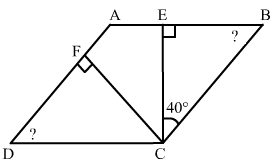Solution:

In triangle CEB: ∠ECB + ∠CBE + ∠BEC = 180° (angle sum property of a triangle)

40° + 90° + ∠EBC = 180°

Therefore, ∠EBC = 50°

Also, ∠EBC = ∠ADC = 50° (opposite angle of a parallelogram)

ln triangle FDC: ∠FDC + ∠DCF + ∠DCF = 180°

50° + 90° + ∠DCF = 180°

Therefore, ∠DCF = 40°

Now, ∠BCE + ∠ECF + ∠FCD + ∠FDC = 180° (in a parallelogram, the sum of alternate angle is 180°

50° + 40° + ∠ECF + 40°=180°

∠ECF = 180° – 50° + 40° – 40° = 50°

Question: 21

The angle between the altitudes of a parallelogram, through the same vertex of an obtuse angle of the parallelogram is 60°. Find the angles of the parallelogram.Solution:

Draw a parallelogram ABCD.

Drop a perpendicular from B to the side AD, at the point E.

Drop a perpendicular from B to the side CD, at the point F.

In the quadrilateral BEDF: ∠EBF = 60°, ∠BED = 90°, ∠BFD = 90°

∠EDF = 360° – (60° + 90° + 90°) = 120°

In a parallelogram, opposite angles are congruent and adjacent angles are supplementary.

In the parallelogram ABCD: ∠B = ∠D = 120°

∠A = ∠C = 180° – 120° = 60°

Question: 22

In Figure, ABCD and AEFG are parallelograms. If ∠C = 55°, what is the measure of ∠F?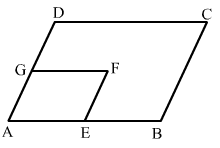Solution:

Both the parallelograms ABCD and AEFG are similar.

Therefore, ∠C = ∠ A = 55° (opposite angles of a parallelogram are equal)

Therefore, ∠A = ∠F = 55° (opposite angles of a parallelogram are equal).

Question: 23

In Figure, BDEF and DCEF are each a parallelogram. Is it true that BD = DC? Why or why not?Solution:

In parallelogram BDEF

Therefore, BD = EF ………..(i) (opposite sides of a parallelogram are equal)

In parallelogram DCEF

CD = EF ……………………(ii) (opposite sides of a parallelogram are equal)

From equations (i) and (ii)

BD = CD

Question: 24

In Figure, suppose it is known that DE = DF. Then, is triangle ABC isosceles? Why not?Solution:

In ΔFDE: DE = DF

∠FED = ∠DFE ………. (i) (angles opposite to equal sides)

In the ||gm BDEF: ∠FBD = ∠FED …….. (ii) (opposite angles of a parallelogram are equal)

In the ||gm DCEF: ∠DCE = ∠DFE ……….. (iii) (opposite angles of a parallelogram are equal)

From equations (i), (ii) and (iii): ∠FBD = ∠DCE

In triangle ABC: if ∠FBD = ∠DCE, then AB = AC (sides opposite to the equal angles.)

Hence, triangle ABC is isosceles.

Question: 25

Diagonals of parallelogram ABCD intersect at O as shown in Figure. XY contain, O, and X, Y are points on opposite sides of the parallelogram. Give reasons for each of the following: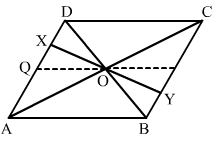(i) OB = OD

(ii) ∠OBY = ∠ODX

(iii) ∠BOY = ∠DOX

(iv) ΔBOY ≅ ΔDOX

Now, state if XY is bisected at O

Solution:

(i) Diagonals of a parallelogram bisect each other.

(ii) Alternate angles

(iii) vertically opposite angles

(iv) ΔBOY and ΔDOX: OB = OD (diagonals of a parallelogram bisect each other)

∠OBY = ∠ODX (alternate angles)

∠BOY = ∠DOX (vertically opposite angles)

ASA congruence:

XO = YO (c.p.c.t)

So, XY is bisected at O.

Question: 26

In figure, ABCD is a parallelogram, CE bisects ∠C and AF bisects ∠A. In each of the following, if the statement is true, give a reason for the same: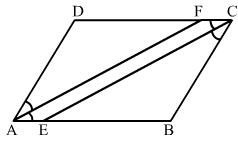(i) ∠A = ∠C

(ii) ∠FAB = 1/2∠A

(iii) ∠DCE = 1/2∠C

(iv) ∠CEB = ∠FAB

(v) CE || AF

Solution:

(i) True, since opposite angles of a parallelogram are equal.

(ii) True, as AF is the bisector of ∠A.

(iii) True, as CE is the bisector of ∠C.

(iv) True

∠CEB = ∠DCE ………….(i) (alternate angles)

∠DCE = ∠FAB ………….(ii) (opposite angles of a parallelogram are equal)

From equations (i) and (ii):

∠CEB = ∠FAB

(v) True, as corresponding angles are equal (∠CEB = ∠FAB).

Question: 27

Diagonals of a parallelogram ABCD intersect at O. AL and CM are drawn perpendiculars to BD such that L and M lie on BD. Is AL = CM? Why or why not?Solution:

In ΔAOL and ΔCMO:

∠AOL = ∠COM (vertically opposite angle)…….(i)

∠ ALO = ∠CM0 = 90° (each right angle)……….. (ii)

Using angle sum property: ∠A0L + ∠ALO + ∠LA0 = 180° …………. (iii)

∠COM + ∠CMO + ∠OCM = 180°………. (iv)

From equations (iii) and (iv):

∠AOL + ∠ALO + ∠LAO = ∠COM + ∠CMO + ∠OCM

∠LAO = ∠OCM (from equation (i) and (ii))

In ΔAOL and ΔCMO:

∠ALO = ∠CMO (each right angle)

AO = OC (diagonals of a parallelogram bisect each)

∠LAO = ∠OCM (proved above)

So, ΔAOL is congruent to ΔCMO (SAS).

AL = CM [CPCT]

Question: 28

Points E and F lie on diagonal AC of a parallelogram ABCD such that AE = CF. What type of quadrilateral is BFDE?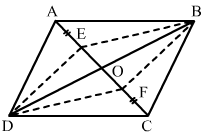Solution:

In the ||gm ABCD:

AO = OC…………. (i) (diagonals of a parallelogram bisect each other)

AE = CF……….(ii) (given)

Subtracting (ii) from (i): AO – AE = OC – CF

EO = OF…………(iii)

In ΔDOE and ΔBOF: EO = OF (proved above)

DO = OB (diagonals of a parallelogram bisect each other)

∠DOE = ∠BOF (vertically opposite angles)

By SAS congruence: ΔDOE ≅ ΔBOF

Therefore, DE = BF (c.p.c.t)

In ΔBOE and ΔDOF:

EO = OF (proved above)

DO = OB (diagonals of a parallelogram bisect each other)

∠DOF = ∠BOE (vertically opposite angles)

By SAS congruence: ΔDOE ≅ ΔBOF

Therefore, DF = BE (c.p.c.t)

Hence, the pair of opposite sides are equal. Thus, DEBF is a parallelogram.

Question: 29

In a parallelogram ABCD, AB = 10 cm, AD = 6 cm. The bisector of ∠A meets DC in E, AE and BC produced meet at F. Find the length CF.

Solution:

AE is the bisector of ∠DAE = ∠BAE = x

∠BAE = ∠AED = x (alternate angles)

Since opposite angles in triangle ADE are equal, Triangle ADE is an isosceles triangle.

Therefore, AD = DE = 6 cm (sides opposite to equal angles)AB = CD = 10 cm

CD = DE + EC

EC = CD –DE

EC = 10 – 6 = 4 cm

∠DEA = ∠CEF = x (vertically opposite angle)

∠EAD = ∠EEFC = x (alternate angles)

Since opposite angle in triangle EFC are equal, Triangle EFC is an isosceles triangle.

Therefore, CF = CE = 4 cm (sides opposite to equal angles)

∴ Therefore CF = 4 cm.
```### Course Features

• 728 Video Lectures
• Revision Notes
• Previous Year Papers
• Mind Map
• Study Planner
• NCERT Solutions
• Discussion Forum
• Test paper with Video Solution